# Formula editor

The Dewesoft formula mathematics enables the user to use a formula parser with algebraic, logic, trigonometric and other functions to recalculate one or several input channels.

When you choose Dewesoft Math Formula, the icon below is displayed: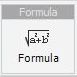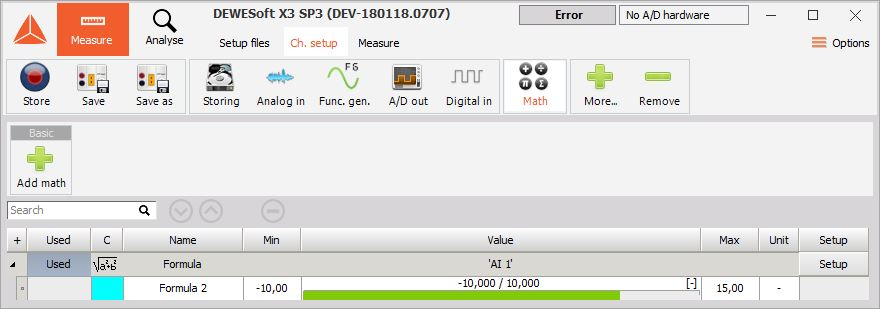For detailed information about basic settings see -> Setup screen and basic operation.

## Formula setup

When you press the Setup button in SETUP column of Math setup screen, the window will appear depending on the selected module.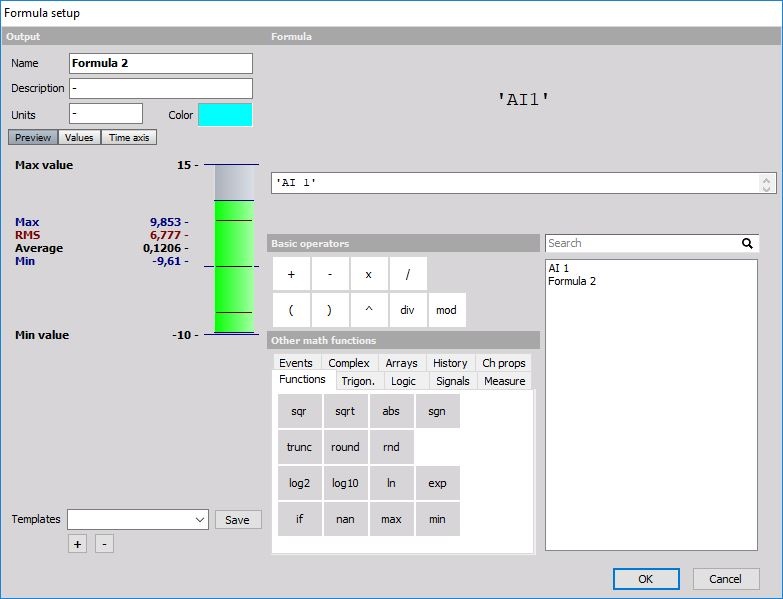The left side of the display is standard display setup, described in Setup screen -> see -> Setup screen and basic operation. The only difference is the sample rate divider (see below), which can be defined for the output channel of the formula. The right side shows the formula editor.

### Sample rate divider

SR div is the sample rate divider of the channel with several options: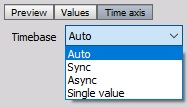• Auto - The sample rate divider will be assigned automatically. For example:
• If the input is a synchronous channel with Sr div of 4, the resulting channel will also have a sample rate divider of four.
• If the input is an asynchronous channel, the output will also be an asynchronous channel.
• If there are more input channels, the output will be matched to the input channel with the highest rate.
• Sync - This function will override the logic of automatic Sr dDiv assignment and will set the sample rate divider manually. This is useful to make interpolation of input asynchronous channel because each asynchronous channel is interpolated before it is calculated. This gives a smoother curve and more precise results.
• Async - This function enables you to set the sample rate of the mathematical formula to the same as the selected asynchronous channel.
• Single value - The result of mathematics is only a single value. Single value is a special type of Dewesoft channel where the result is not a set of values with a time stamp, but always just one value. This is useful for calculating cumulative values. Sync (From the list, we can select Manual values from 1 to 500).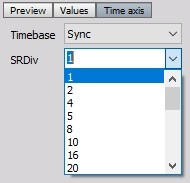Async (In channel the drop-down menu, you can select the appropriate trigger).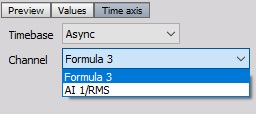### Formula editor

The right side, “Formula” part of the Formula setup window contains: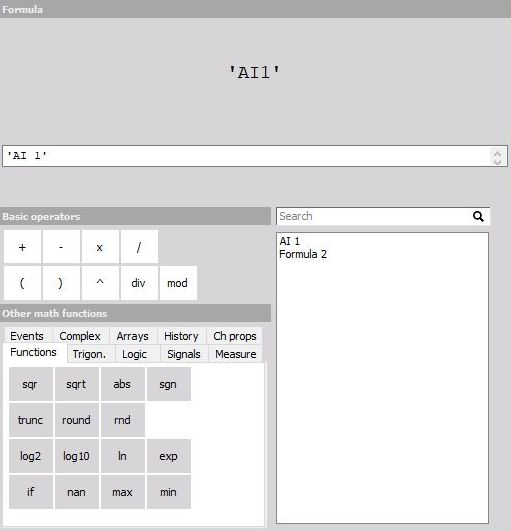• a formula preview at the top
• the field to enter the formula directly
• the mathematical functions and operators with:
• Basics operators
• Other math functions (Functions, Logic, Trigonometry, Signals, Measure, Events)
• list of all available channels: analog channels, variables, constants

To enter a channel in the formula just:

• double-click on the channel (in list of all channels)
• single-click on the function (in ‘Other math functions’)
• single-click on the operator (In ‘Basic operators’)

Dewesoft allows doing mathematics on mathematics in any combination. The Formula interpreter allows also enter complex formulas.

If you do a mathematical operation on two channels with the different sampling rate, the two channels will be synchronized to each other. The values of the slower sampled signal will be repeated for the calculation with the faster-sampled signal until a new value is available.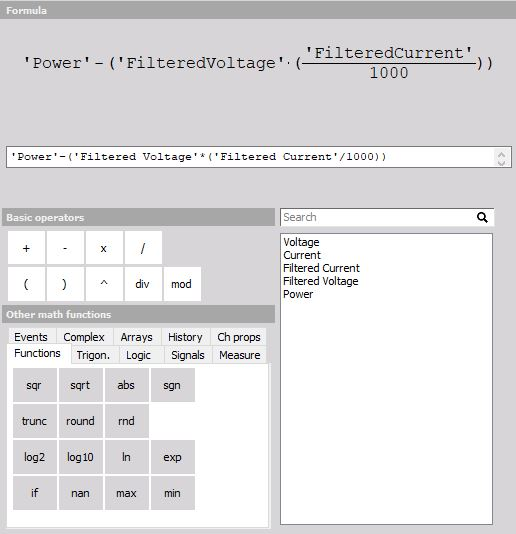All available channels are displayed in the selection list. For Dewesoft it makes no difference where the data are coming from: analog input, CAN, mathematics, GPS,… - even different sampling rates are no problem.

Dewesoft allows different types of mathematics, which are grouped in six tabs. Please learn more about these functions in -> Operators and mathematical function.

If you make an error, you will get an indicator which shows you immediately where the error is located and error description.

Some error examples: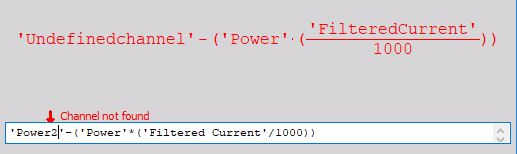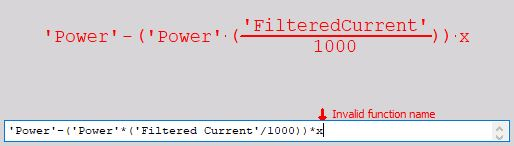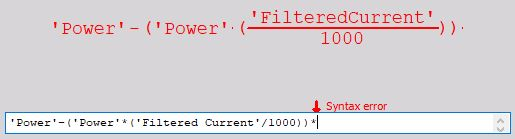When you have entered your formula, press the OK button to leave the setup. Now a new line has been added to the mathematics overview screen: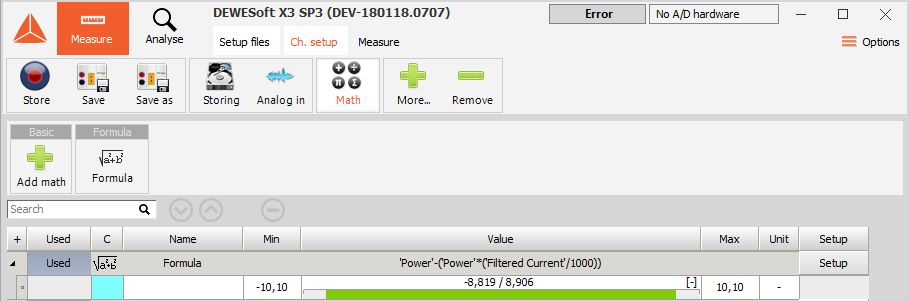See next topic to have a complete list of available operators and mathematical functions.